# How to plot a tri-variate data distribution in 3D and add surfaces of confidence ellipsoids from a trivariate normal distribution with the same covariance matrix

This post requires Javascript to display formulas!

In Machine Learning [ML] or statistics it is interesting to visualize properties of multivariate distributions by projecting them into 2- or 3-dimensional sub-spaces of the datas’ original n-dimensional variable space. The 3-dimensional aspects are not so often used because plotting is more complex and you have to fight with transparency aspects. Nevertheless a 3-dim view on data may sometimes be more instructive than the analysis of 2-dim projections. In this post we care about 3-dim data representations of tri-variate distributions X with matplotlib. And we add ellipsoids from a corresponding tri-variate normal distribution with the same covariance matrix as X.

# Multivariate normal distribution and their projection into a 3-dimensional sub-space

A statistical multivariate distribution of data points is described by a so called random vector X in an Euclidean space for the relevant variables which characterize each object of interest. Many data samples in statistics, big data or ML are (in parts) close to a so called multivariate normal distribution [MND]. One reason for this is, by the way, the “central limit theorem”. A multivariate data distribution in the ℝn can be projected orthogonally onto a 3-dimensional sub-space. Depending on the selected axes that span the sub-space you get a tri-variate distribution of data points.

Whilst analyzing a multivariate distribution you may want to visualize for which regions of variable values your projected tri-variate distributions X deviate from adapted and related theoretical tri-variate normal distributions. The relation will be given by relevant elements of the covariance matrix. Such a deviation investigation defines an application “case 1”.

Another application case, “case 2”, is the following: We may want to study a 3-variate MND, a TND, to get a better idea about the behavior of MNDs in general. In particular you may want to learn details about the relation of the TND with its orthogonal projections onto coordinate planes. Such projections give you marginal distributions in sub-spaces of 2 dimensions. The step from analyzing bi-variate to analyzing tri-variate normal distributions quite often helps to get a deeper understanding of MNDs in spaces of higher dimension and their generalized properties.

When we have a given n-dimensional multivariate random vector X (with n > 3) we get 3-dimensional data by applying an orthogonal projection operator P on the vector data. The relatively trivial operator projects the data orthogonally into a sub-volume spanned by three selected axes of the full variable space. For a given random vector their will, of course, exist multiple such projections as there is a whole bunch of 3-dim sub-spaces for a big n. In “case 2”, however, we just create basic vectors of a 3-dim MND via a proper random generation function.

Regarding matplotlib for Python we can use a scatter-plot function to visualize the resulting data points in 3D. Typically, plots of an ideal or approximate tri-variate normal distribution [TND] will show a dense ellipsoidal core, but also a diffuse and only thinly populated outer region. To get a better impression of the spatial distribution of X relative to a TND and the orientation of the latter’s main axes it might be helpful to include ideal contour surfaces of the TND into the plots.

It is well known that the contour surfaces of multivariate normal distributions are surfaces of nested ellipsoids. On first sight it may, hower, appear difficult to combine impressions of such 2-dim hyper-surfaces with a 3-dim scatter plot. In particular: Where from do we get the main axes of the ellipsoids? And how to plot their (hyper-) surfaces?

# Objective of this post

The objective of this post is to show that we can derive everything that is required

• to plot general tri-variate distributions
• plus ellipsoids from corresponding tri-variate normal distributions

from the covariance matrix Σ of our random vector X.

In case 2 we will just have to define such a matrix – and everything else will follow from it. In case 1 you have to first determine the (n x n)-covariance matrix of your random vector and then extract the relevant elements for the (3 x 3)-covariance matrix of the projected distribution out of it.

The result will be plots like the following: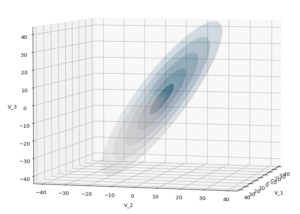The first plot combines a scatter plot of a TND with ellipsoidal contours. The 2nd plot only shows contours for confidence levels 1 ≤ σ ≤ 5 of our ideal TND. And I did a bit of shading.

How did I get there?

# The covariance matrix determines everything

The mathematical object which characterizes the properties of a MND is its covariance matrix (Σ). Note that we can determine a (n x n)-covariance matrix for any X in n dimensions. Numpy provides a function cov() that helps you with this task. The relevant elements of the full covariance matrix for orthogonal projections into a 3-dim sub-space can be extracted (or better: cut out) by applying a suitable projection operator. This is trivial: Just select the elements with the (i, i)- and (i, j)-indices corresponding to the selected axes of the sub-space. The extracted 9 elements will then form the covariance matrix of the projected tri-variate distribution.

In case 2 we just define a (3 x 3)-Σ as the starting point of our work.

Let us assume that we got the essential Σ-matrix from an analysis of our distribution data or that we, in case 2, have created it. How does a (3 x 3)-Σ relate to ellipsoidal surfaces that show the same deformation and relations of the axes’ lengths as a corresponding tri-variate normal distribution?

# Creation of a MND from a standardized normal distribution

In general any n-dim MND can be constructed from a standardized multivariate distribution of independent (and consequently uncorrelated) normal distributions along each axis. I.e. from n univariate marginal distributions. Let us call the standardized multivariate distribution SMND and its random vector Z. We use the coordinate system [CS] where the coordinate axes are aligned with the main axes of the SMND as the CS in which we later also will describe our given distribution X. Furthermore the origin of the CS shall be located such that the SMND is centered. I.e. the mean vector μ of the distribution shall coincide with the CS’s origin:

$\pmb{\mu} \: = \: \pmb{0}$

In this particular CS the (probability) density function f of Z is just a product of Gaussians gj(zj) in all dimensions with a mean at the origin and standard deviations σj = 1, for all j.

With z = (z1, z2, …, zn) being a position vector of a data point in the distribution, we have:

\begin{align} \pmb{\mu} \: &= \: \pmb{0} \\ f_{\pmb{Z}} \:&=\: \pmb{\mathcal{N}}_n\left(\pmb{0}, \, \pmb{\operatorname{I}}_n \right) \:=\: \pmb{\mathcal{N}}_n\left(\pmb{\mu}=0 ,\, \pmb{\operatorname{\Sigma}}=\pmb{\operatorname{I}}_n \right) \end{align}

and in more detail

\begin{align} f_{\pmb{Z}}(\pmb{z}) \, =\, \pmb{\mathcal{N}}_n\left(\pmb{0}, \, \pmb{\operatorname{I}}_n \right) \: &= \: \prod\limits_{j=1}^n g(z_j, \, \mu_j=0, \, \sigma_j=1) \\ &= \, {1 \over \sqrt{(2\pi)^n} } \, {\large e}^{ – \, {\Large 1 \over \Large 2} {\Large \sum\limits_{j=1}^n} \, {\Large z_j}^2 } \\ &=\, {1 \over \sqrt{(2\pi)^n} } \, {\large e}^{ – \, {\Large 1 \over \Large 2} {\Large \pmb{z}^T\pmb{z}} } \end{align}

The construction recipe for the creation of a general MND XN from Z is just the application of a (non-singular) linear transformation. I.e. we apply a (n x n)-matrix onto the position vectors of the data points in the ℝn. Let us call this matrix A. I.e. we transform the random vector Z to a new random vector XN by

$\pmb{X}_N \: = \: \pmb{\operatorname{A}} \circ \pmb{Z}$

The resulting symmetric (!) covariance matrix ΣX of XN is given by

$\pmb{\operatorname{\Sigma}}_X \: = \: \pmb{\operatorname{A}} \pmb{\operatorname{A}}^T$

Σ determines the shape of the resulting probability distribution completely. We can reconstruct an A’ which produces the same distribution by an eigendecomposition of the matrix ΣX. A’ afterward appears as a combination of a rotation and a scaling. An eigendecomposition leads in general to

$\pmb{\operatorname{\Sigma}}_{X_N} \: = \: \pmb{\operatorname{V}} \pmb{\operatorname{D}} \pmb{\operatorname{V}}^{-1}$

with V being an orthogonal matrix and D being a diagonal matrix. In case of a symmetric, positive-definite matrix like our Σ we even can get

$\pmb{\operatorname{\Sigma}}_{X_N} \: = \: \pmb{\operatorname{V}} \pmb{\operatorname{D}} \pmb{\operatorname{V}}^T$

D contains the eigenvalues of Σ, whereas the columns of V are the components of the eigenvectors of Σ (in the present coordinate system). V represents a rotation and D a scaling.

The required transformation matrix T, which leads from the unrotated and unscaled SMND Z to the MND XN, can be rewritten as

$\pmb{\operatorname{A}}’ \: = \: \pmb{\operatorname{T}} \: = \: \pmb{\operatorname{V}} \pmb{\operatorname{D}}^{1/2}$

with

$\pmb{\operatorname{\Sigma}}_{X_N} \: = \: \pmb{\operatorname{A}}’ \left(\pmb{\operatorname{A}}’\right)^T$

The 1/2 abbreviates the square root of the matrix values (i.e. of the eigenvalues). A relevant condition is that ΣX is a symmetric and positive-definite matrix. Meaning: The original A itself must not be singular!

This works in n dimensions as well as in only 3.

# Creation of a trivariate normal distribution

The creation of a centered tri-variate normal distribution is easy with Python and Numpy: We just can use

np.random.multivariate_normal( mean, Σ, m )

to create m statistical data points of the distribution. Σ must of course be delivered as a (3 x 3)-matrix – and it has to be positive definite. In the following example I have used

$\pmb{\operatorname{\Sigma}}_X \:=\: {\begin{pmatrix} 31 & -4 & 5 \\ -4 & 26 & 44 \\ 5 & 44 & 85 \end{pmatrix}}$

We have to feed this Σ-matrix directly into np.random.multivariate_normal(). The result with 20,000 data points looks like: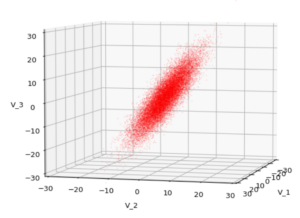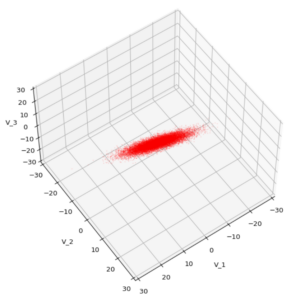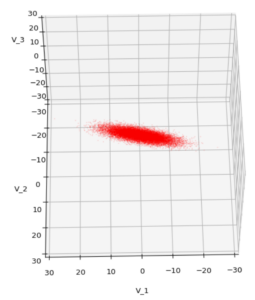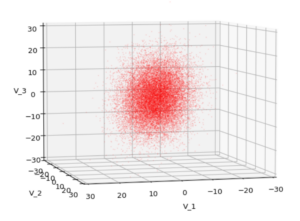We see a dense inner core, clearly having an ellipsoidal shape. While the first image seems to indicate an overall slim ellipsoid, the second image reveals that our TND actually looks more like an extended lens with a diagonal orientation in the CS. =>
Note: When making such §D-plots we have to be careful not to make premature conclusions about the overall shape of the distribution! Projection effects may give us a wrong impression when looking from just one particular position and viewing angle onto the 3-dimensional distribution.

# Create some nested transparent ellipsoidal surfaces

Especially in the outer regions the distribution looks a bit fluffy and not well defined. The reason is the density of points drops rapidly beyond a 3-σ-level. One gets the idea that a sequence of nested contour surfaces may be helpful to get a clearer image of the spatial distribution. We know already that contour surfaces of a MND are the surfaces of ellipsoids. How to get these surfaces?

The answer is rather simple: We just have to apply the mathematical recipe from above to specific data points. These data points must be located on the surface of a unit sphere (of the Z-distribution). Let us call an array with three rows for x-, y-, z-values and m columns for the amount of data points on the 3-dimensional unit sphere US. Then we need to perform the following transformation operation:

$\pmb{S}_X \: = \: \pmb{\operatorname{T}} \circ \pmb{US}$

to get a valid distribution SX of points on the surface of an ellipsoid with the right orientation and relation of the main axes lengths. The surface of such an ellipsoid defines a contour surface of a TND with the covariance matrix Σ.

After the application of T to these special points we can use matplotlib’s

ax.plot_surface(x, y, z, …)

to get a continuous surface image.

To get multiple nested surfaces with growing diameters of the related ellipsoids we just have to scale (with growing and common integer factors applied to the σj-eigen-values).

Note that we must create all the surfaces transparent – otherwise we would not get a view to inner regions and other nested surfaces. See the code snippets below for more details.

# SVD decomposition

We also have to get serious about numerically calculating T. I.e., we need a way to perform the “eigendecomposition”. Technically, we can use the so called “Singular Value Decomposition” [SVD] from Numpy’s linalg-module, which for symmetric matrices just becomes the eigendecomposition.

# Code snippets

We can now write down some Jupyter cells with Python code to realize our SVD decomposition. I omit any functionality to project your real data into a 3-dim sub-space and to derive the covariance matrix. These are standard procedures in ML or statistics. But the following code snippets will show which libraries you need and the basic steps to create your plots. Instead of a general MND, I will create a TND for scatter plot points:

Code cell 1 – Imports

import numpy as np
import matplotlib as mpl
from matplotlib import pyplot as plt
from matplotlib.colors import ListedColormap
import matplotlib.patches as mpat
from matplotlib.patches import Ellipse
from matplotlib.colors import LightSource


Code cell 2 – Function to create points on a unit sphere

# Function to create points on unit sphere
def pts_on_unit_sphere(num=200, b_print=True):

# Create pts on unit sphere
u = np.linspace(0, 2 * np.pi, num)
v = np.linspace(0, np.pi, num)

x = np.outer(np.cos(u), np.sin(v))
y = np.outer(np.sin(u), np.sin(v))
z = np.outer(np.ones_like(u), np.cos(v))

# Make array
unit_sphere = np.stack((x, y, z), 0).reshape(3, -1)

if b_print:
print()
print("Shapes of coordinate arrays : ", x.shape, y.shape, z.shape)
print("Shape of unit sphere array : ",  unit_sphere.shape)
print()

return unit_sphere, x


Code cell 3 – Function to plot TND with ellipsoidal surfaces

# Function to plot a TND with supplied allipsoids based on the sam Sigma-matrix
def plot_TND_with_ellipsoids(elevation=5, azimuth=5,
size=12, dpi=96,
lim=30, dist=11,
X_pts_data=[],
li_ellipsoids=[],
li_alpha_ell=[],
b_scatter=False, b_surface=True,
b_antialias=True,
pts_size=0.02, pts_alpha=0.8,
uniform_color='b', strid=1,
light_azim=65, light_alt=45
):

# Prepare figure
plt.rcParams['figure.dpi'] = dpi
fig = plt.figure(figsize=(size,size))  # Square figure

# Check data
if b_scatter:
if len(X_pts_data) == 0:
print("Error: No scatter points available")
return

if b_surface:
if len(li_ellipsoids) == 0:
print("Error: No ellipsoid points available")
return
if len(li_alpha_ell) < len(li_ellipsoids):
print("Error: Not enough alpha values for ellipsoida")
return
num_ell = len(li_ellipsoids)

# set limits on axes
if b_surface:
lim = lim * 1.7
else:
lim = lim

# axes and labels
ax.set_xlim(-lim, lim)
ax.set_ylim(-lim, lim)
ax.set_zlim(-lim, lim)

# scatter points of the MND
if b_scatter:
x = X_pts_data[:, 0]
y = X_pts_data[:, 1]
z = X_pts_data[:, 2]
ax.scatter(x, y, z, c='r', marker='o', s=pts_size, alpha=pts_alpha)

# ellipsoidal surfaces
if b_surface:
# Light source
ls = LightSource(azdeg=light_azim, altdeg=light_alt)
cm = plt.cm.PuBu
li_rgb = []
for i in range(0, num_ell):
zz = li_ellipsoids[i][2,:]

if b_common_rgb:
for i in range(0, num_ell-1):
li_rgb[i] = li_rgb[num_ell-1]

# plot the ellipsoidal surfaces
for i in range(0, num_ell):
ax.plot_surface(*li_ellipsoids[i],
rstride=strid, cstride=strid,
linewidth=0, antialiased=b_antialias,
facecolors=li_rgb[i],
alpha=li_alpha_ell[i]
)
# just uniform color
else:
print("here")
#ax.plot_surface(*ellipsoid, rstride=2, cstride=2, color='b', alpha=0.2)
for i in range(0, num_ell):
ax.plot_surface(*li_ellipsoids[i],
rstride=strid, cstride=strid,
color=uniform_color, alpha=li_alpha_ell[i])

ax.view_init(elev=elevation, azim=azimuth)
ax.dist = dist
return


Some hints: The scatter data from the distribution X (or XN) must be provided as a Numpy array. The TND-ellipsoids and the respective alpha values must be provided as Python lists. Also the size and the alpha-values for the scatter points can be controlled. Reducing both can be helpful to get a glimpse also on ellipsoids within the relative dense core of the TND.

Some parameters as “elevation”, “azimuth”, “dist” help to control the viewing perspective. You can switch showing of the scatter data as well as ellipsoidal surfaces and their shading on and off by the Boolean parameters.

There are a lot of parameters to control a primitive kind of shading. You find the relevant information in the online documentation of matplotlib. The “strid” (stride) parameter should be set to 1 or 2. For slower CPUs one can also take higher values. One has to define a light-source and a rgb-value range for the z-values. You are free to use a different color-map instead of PuBu and make that a parameter, too.

Code cell 4 – Covariance matrix, SVD decomposition and derivation of the T-matrix

b_print = True

# Covariance matrix
cov1 = [[31.0, -4, 5], [-4, 26, 44], [5, 44, 85]]
cov = np.array(cov1)

# SVD Decomposition
U, S, Vt = np.linalg.svd(cov, full_matrices=True)
S_sqrt = np.sqrt(S)

# get the trafo matrix T = U*SQRT(S)
T = U * S_sqrt

# Print some info
if b_print:
print("Shape U: ", U.shape, " :: Shape S: ", S.shape)
print(" S      : ", S)
print(" S_sqrt : ", S_sqrt)
print()
print(" U :\n", U)
print(" T :\n", T)


Code cell 5 – Transformation of points on a unit sphere

# Transform pts from unit sphere onto surface of ellipsoids
# ~~~~~~~~~~~~~~~~~~~~~~~~~~~~~~~
b_print = True
num = 200
unit_sphere, xs = pts_on_unit_sphere(num=num)

# Apply transformation on data of unit sphere
ell_transf = T @ unit_sphere
# li_fact = [2.5, 3.5, 4.5, 5.5, 6.5]
li_fact = [1.0, 2.0, 3.0, 4.0, 5.0]
num_ell = len(li_fact)
li_ellipsoids = []
for i in range(0, num_ell):
li_ellipsoids.append(li_fact[i] * ell_transf.reshape(3, *xs.shape))

if b_print:
print("Shape ell_transf  : ", ell_transf.shape)
print("Shape ell_transf0 : ", li_ellipsoids.shape)


Code cell 6 – TND data point creation and plotting

# Create TND-points
n = 10000
mean=[0,0,0]
pts_data = np.random.multivariate_normal(mean, cov1, n)

# Alpha values for the ellipsoids
common_alpha = 0.05
li_alpha_ell = []
for i in range(0, num_ell):
li_alpha_ell.append(common_alpha)
#li_alpha_ell = [0.08, 0.05, 0.05, 0.05, 0.05]
#li_alpha_ell = [0.12, 0.1, 0.08, 0.08, 0.08]
li_alpha_ell = [0.3, 0.1, 0.08, 0.08, 0.08]

# Plotting
elev = 5
azim = 15
plot_TND_with_ellipsoids(elevation=elev, azimuth=azim,
lim= 25, dist=11,
X_pts_data = pts_data,
li_ellipsoids=li_ellipsoids,
li_alpha_ell=li_alpha_ell,
b_scatter=True, b_surface=True,
b_antialias=True,
pts_size=0.018, pts_alpha=0.6,
uniform_color='b', strid=2,
light_azim=65, light_alt=75
)
#


# Example views

Let us play a bit around. The ellipsoidal contours are for σ = 1, 2, 3 ,4, 5. First we look at the data distribution from above. The ellipsoidal contours are for σ = 1, 2, 3 ,4, 5. We must reduce the size and alpha of the scatter points to 0.01 and 0.45, respectively, to still get an indication of the innermost ellipsoid of σ = 1. I used 8000 scatter points. The following plots show the same from different side perspectives – with and without shading and adapted alpha-values and number values for the scatter points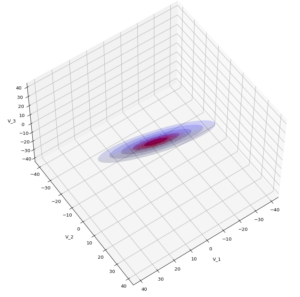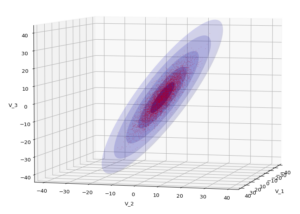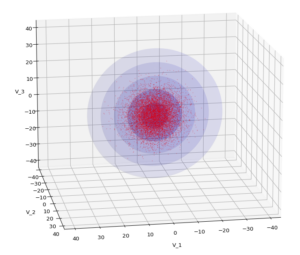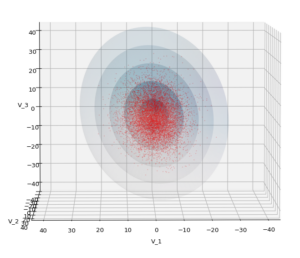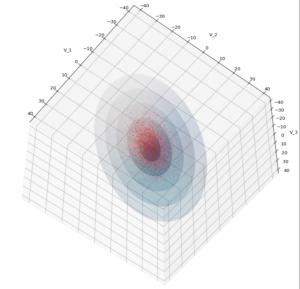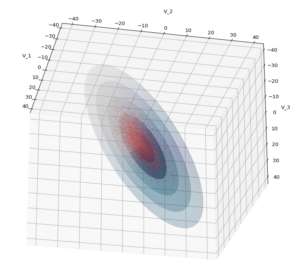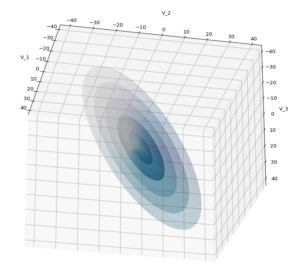Yoo see: How a TND appears depends strongly on the viewing position. From some positions the distribution’s projection of the viewer’s background plane may even appear spherical. But on the other side it is remarkable how well the projection onto a background plane keeps up the ellipses of the projected outermost borders of the contour surfaces (of the ellipsoids for confidence levels). This is a dominant feature of MNDs in general.

A last hint: To get a more volumetric impression it is required to both work with the position of the lightsource, the alpha-values and the colormap. In addition turning off ant-aliasing and setting the stride to something like 5 may be very helpful, too. The next image was done for an ellipsoid with slightly different extensions, only 3 ellipsoids and stride=5: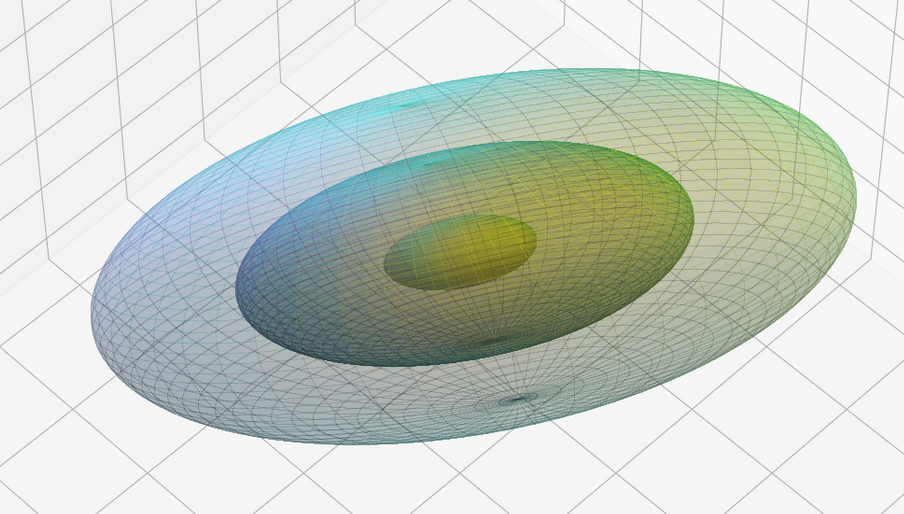# Conclusion

In this blog I have shown that we can display a tri-variate (normal) distribution, stemming from a construction of a standardized normal distribution or from a projection of some real data distribution, in 3D with the help of Numpy and matplotlib. As soon as we know the covariance matrix of our distribution we can add transparent surfaces of ellipsoids to our plots. These are constructed via a linear transformation of points on a unit sphere. The transformation matrix can be derived from an eigendecomposition of the covariance matrix.

The added ellipsoids help to better understand the shape and orientation of the tri-variate distribution. But plots from different viewing angles are required.

# Multivariate Normal Distributions – I – objectives

This post requires Javascript to display formulas!

Machine Learning [ML] algorithms are applied to multivariate data: Each individual object of interest (e.g. an image) is characterized by a set of n distinct and quantifiable variables. The variable values may e.g. come from measurements.

A sample of such objects corresponds to a data distribution in a multidimensional space, most often the ℝn. We can visualize our objects as data points in an Euclidean coordinate system of the ℝn: Each axis represents the values a specific variable can take; the position of a data point is given by the variable values.

Equivalently, we can use (position-) vectors to these data points. Thus, when training ML algorithms we typically deal with vector distributions, which by their very nature are multivariate. But also the outputs of some types of neural networks like Autoencoders [AE] form multivariate distributions in the networks’ latent spaces. For today’s ML-scenarios the number of dimensions n can become very big – even if we compress information in latent spaces. For a variety of tasks in generative ML we may need to understand the nature and shape of such distributions.

An elementary kind of a continuous multivariate vector distribution, for which major properties can be derived analytically, is the so called Multivariate Normal Distribution [MND]. MNDs, their marginal and their conditional distributions are of major importance both in the fields of statistics, Big Data and Machine Learning. One reason for this is the “central limit theorem” of statistics (in its vector form).

Some conventional ML-algorithms are even based on the assumption that the population behind the concrete data samples can be approximated by a MND. Due to the central limit theorem we find that averages of big samples of multivariate training data for a population of specific types of observed objects tend to form a MND. But also data samples in latent spaces of neural networks may show a multivariate normal distribution – at least in parts.

For the concrete problem of human face generation via a trained convolutional Autoencoder [CAE] I have actually found that the data produced in the CAE’s latent space can very well be described by a MND. See the posts on Autoencoders in this blog. This alone is motivation enough to dive a bit deeper into the (beautiful) mathematical properties of MNDs.

Just to illustrate it: The following plots show projections of the approximate MND onto coordinate planes.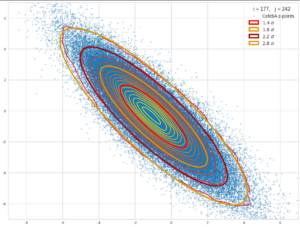We find the typical elliptic contour lines which are to be expected for a MND. And here are some generated face images from statistical vectors which I derived from an analysis of the characteristic features of the 2-dim projections of the latent MND which my CAE had produced: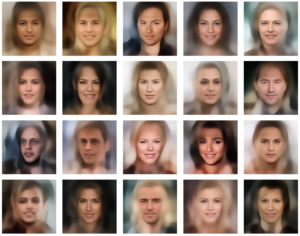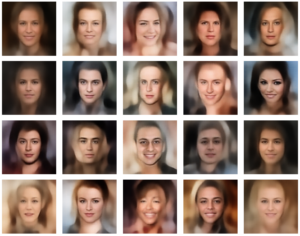# ML is math in the end – and MNDs are no exception

Some of my readers may have noticed that I wanted to start a series on the topic of creating random vectors for a given MND-like vector distribution. The characteristic parameters for the n-dimensional MND can either stem from an analysis of experimental ML data or come from theoretical sources. This was in April. But, I have been silent on this topic for a while.

The reason was that I got caught up in the study of the math of MNDs, of their properties, their marginal distributions and of quadratic forms in multiple dimensions (ellipsoids and ellipses). I had to re-collect a lot of mathematical information which I once (45 years ago) had learned at university. Unfortunately, multivariate analysis (i.e. data analysis in multidimensional spaces) requires some (undergraduate) university math. Regarding MNDs, knowledge both in linear algebra, statistics and vector analysis is required. In particular matrices, their decomposition and their geometrical interpretation play a major role. And when you try to understand a particular problem which obviously is characterized by an overlap of multiple mathematical disciplines the amount of information can quickly grow – without the connections and consistency becoming clear at first sight.This is in part due to the different fields the authors of papers on MNDs work in and the different focuses they have on properties of MNDs. Although many introductory information about MNDs is available on the Internet, I have so far missed a coherent and comprehensive presentation which illustrates the theoretical insights by both ideal and real world examples. Too often the texts are restricted to pure formal derivations. And none of the texts discussed the problem of vector generation within the limits of MND confidence levels. But this task can become important in generative ML: At high confidence levels outliers become a strong weight – and deviations from an ideal MND may cause disturbances.

One problem with appropriate vector generation for creative ML purposes is that ML experiments deliver (latent) data which are difficult to analyze as they reside in high-dimensional spaces. Even if we already knew that they form a MND in some parts of a latent space we would have to perform a drill down to analytic formulas which describe limiting conditions for the components of the statistical vectors we want to create.

The other problem is that we need a solid understanding of confidence levels for a multidimensional distribution of data points, which we approximate by a MND. And on one’s way to understanding related properties of MNDs you pass a lot of interesting side aspects – e.g. degenerate distributions, matrix decompositions, affine transformations and projections of multidimensional hypersurfaces onto coordinate planes. Far too interesting to refrain from not writing something about it …

After having read many publicly available articles on MNDs and related math I had collected a bunch of notes, formulas and numerical experiments. The idea of a general post series on MNDs grew in parallel. From my own experiences I thought that ML people who are confronted with latent representations of data and find indications of a MND would like to have an introduction which covers the most relevant aspects of MNDs. On a certain mathematical level, and supported by illustrations from a concrete example.

But I will not forget about my original objective, namely the generation of random vectors within confidence levels. In the end we will find two possible approaches: One is based on a particular linear transformation, whose mathematical form is determined by a covariance analysis of our data distribution, and random number generators for multiple Gaussian distributions. The other solution is based on a derivation of precise conditions on random vector components from ellipses which are produced by projections of our real experimental data distribution onto coordinate planes. Such limiting conditions can be given in form of analytic expressions.

The second approach can also be understood as a reconstruction of a multivariate distribution from low-dimensional projection data:

We create vectors of a concrete MND-like vector distribution in n dimensions by only referring to characteristic data of its two-dimensional projections onto coordinate planes.

This is an interesting objective in itself as the access to and the analysis of 2-dimensional (correlated) data may be a much easier endeavour than analyzing the full distribution. But such an approach has to be supported by mathematical arguments.

# Objectives of this post series

Objectives of this post series are:

1. We want to find out what a MND is in mathematical and statistical terms and how it can be based on a simpler vector distributions within the ℝn.
2. We want to study the basic role of a standardized multivariate normal distribution in the game and the impact of linear affine transformations on such a distribution – in terms of linear algebra and from a geometrical point of view.
3. We also want to describe and interpret the difference between normal MNDs and so called degenerate MNDs.
4. We want to understand the most important mathematical properties of MNDs. In particular we want to better grasp the mathematical meaning of correlations between the vector components and their impact on the probability density function. Furthermore the relation of a MND to its marginal distributions in sub-spaces of lower dimensions is of major interest.
5. We want to formally create a MND-approximation to a real multivariate data distribution by an analysis of real distribution’s properties and in particular from parameters describing the correlations between the vector components. Of particular interest are the covariance matrix and the precision or correlation matrix.
6. We want to study the role of projections when turning from a MND to its marginal distributions and the impact of such projections on the matrices qualifying the original and its marginal distributions.
7. We want to understand the form of contour hyper-surfaces for constant probability density values of a MND. We also want to derive what the projections of these hyper-surfaces onto coordinate planes look like.
8. We want to show that both contour hyper-surfaces of the MND and of its projections in marginal distributions contain the same proportions of integrated data points and, equivalently, the same probability proportions resulting from an integration of the probability density from the distribution’s center up to the hyper-surfaces.
9. We want to illustrate basic MND-creation principles and the effects of linear affine transformations during the construction process by an ideal 3-dimensional MND example and by projections of a real vector distribution from an ML-experiment onto 2-dimensional and 3-dimensional sub-spaces. We also want to illustrate the relation between the MND and its marginal distributions by plotting concrete 3-dimensional examples and their projections onto coordinate planes.
10. We want to use the derived MND properties for the creation of statistical vectors v which fulfill the following conditions:
• Each of the generated v is a member of a vector population, which has been derived from a ML experiment and which to a good approximation can be described by a MND (and its extracted basic parameters).
• Each v has an endpoint within the multidimensional volume enclosed by a contour-hypersurface of the MND’s probability density function [p.d.f.],
• The limiting hypersurface is defined by a chosen confidence level.
11. We want to create statistical vectors within the limit of contour hyper-surfaces by using elementary construction principles of a MND.
12. In a second approach we want to reduce vector creation to solving a sequence of 2-dimensional problems. I.e. we want to work with 2-dim marginal distributions in 2-dim sub-spaces of the ℝn. We hope that the probability density functions of the relevant distributions can be described analytically and provide computable limiting conditions on vector components.
Note: The production of statistical vectors from data of projected low-dimensional marginal distributions corresponds to a reconstruction of the full MND from its projections.
13. During random vector creation we want to avoid PCA-transformations of the whole real data distribution or of projections of it.
14. Based on MND-parameters we want to find analytic expressions for the vector component limits whenever possible.

The attentive reader has noticed that the list above includes an assumption – namely that a multidimensional contour hypersurface of a MND can be associated with something like a confidence level. In addition we have to justify mathematically that the reduction to data of 2-dimensional projections of the full vector distribution is a real option for statistical vector creation.

The last three points are a bit tough: Even if we believe in math textbooks and get limiting hyper-curves of a quadratic form in our coordinate planes the main axes of the respective ellipses may show angles versus the coordinate axes (see the example images above). All this would have to be taken care of in a precise analytic form of the limits which we impose on the components of our aspired statistical vectors.

So, this series is, at least in parts, going to be a tough, but also very satisfactory journey. Eventually, after having clarified diverse properties of MNDs and their marginal distributions in lower dimensional spaces, we will end up with quadratic equations and some simple matrix operations.

# Objectives of the next post

We must not forget that statistics plays a major role in our business. In ML we deal with finite collections (samples) of individual object data which are statistically picked from a greater population (with assumed statistical properties). An example is a concrete collection of images of human faces and/or their latent vectors. The data can be organized in form of a two-dimensional data matrix: Its rows may indicate individual objects and its columns properties of these objects (or vice versa). In either direction we have vectors which focus on a particular aspect of the data: Individual objects or the statistics of a specific object property.

While we are used to univariate “random variables” we have to turn to so called “random vectors” to describe multidimensional statistical distributions and respective samples picked from an underlying population. A proper vector notation will give us the advantage of writing down linear transformations of a whole multidimensional vector distribution in a short and concise form.

Besides introducing random vectors and their components the next post

Multivariate Normal Distributions – II – random vectors and their covariance matrix

will also discuss related probability densities, expectation values and the definition of a covariance matrix for a random vector. Some simple properties of the covariance matrix will help us in further posts.